# Using the standard enthalpies of formation, what is the standard enthalpy of reaction?

using the standard enthalpies of formation, what is the standard enthalpy of reaction?

CO(g) +H2O(g) =CO2 (g) + H2 (g)

Concepts and reason
The standard enthalpy of formation:
It is the enthalpy change when one mole of a substance is formed from its pure elements under standard conditions.

Fundamentals
The formula to calculate standard enthalpy of formation is given below: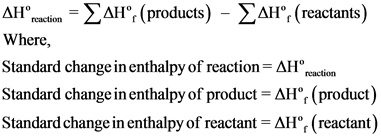The balanced chemical equation is as follows: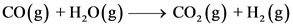The formation values are as follows:Explanation:
The balanced chemical reaction is written and the formation values are indicated for each species.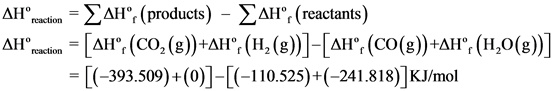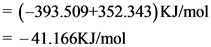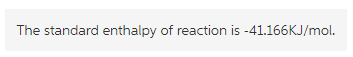Explanation:
The standard enthalpy of reaction is written and the values of standard enthalpies of formation were substituted and solved to get the answer.